True Projections

True projections are projections for which − in case the projection axis is identical with the Pole Axis −

• the images of the longitudes are straight lines,
• the images of the latitudes either are straight lines or (concentric) circles and
• all lines intersect each other rectangularily.

For instance the projections below comply with these conditions. In case of two of these projections the surface of the sphere is first mapped onto an auxiliary surface which then will be unwinded onto the plane. Here we are speaking of the conic and the cylindrical projection. Rotation cones resp. rotation cylinders are developable (auxiliary) surfaces. In case of the azimuthal projection the surface of the sphere is mapped onto the plane directly.

Principle of the Conic ProjectionPrinciple of the Azimuthal Projection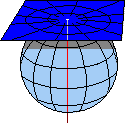Principle of the Cylindrical ProjectionIn the mathematically view the conic projection is the most general case of true projections because of the cylinder and the plane are variations of the cone. A cylinder is a limiting form of a cone with an increasingly sharp point or apex. As the cone becomes flatter, its limit is a plane. Thus the true projections are also called conical projections.

To each of these projections there exists equal-area, equidistant and conformal variants depending on the intended use of the current map. Take a choice and have a look.

Advanced descriptions and explanations are offered in the program  GridMaps .

Conic Projections

Equal-area conic projections (Tissot's Indicatrices with 30° graticule)×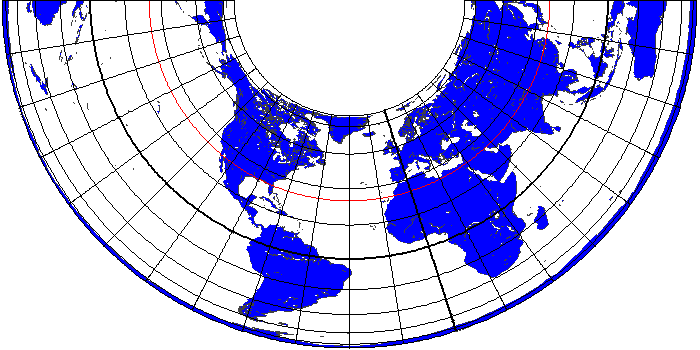×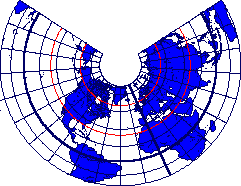×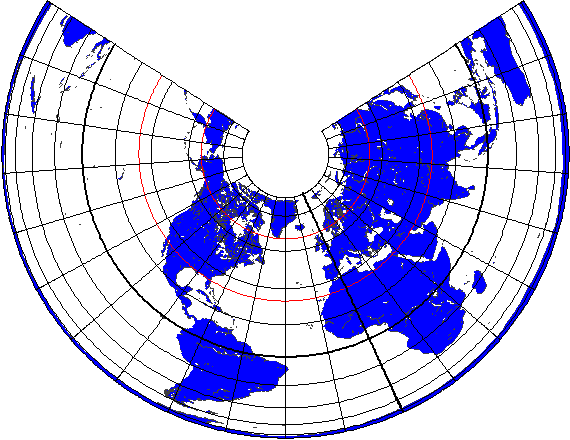×Equidistant conic projections (Tissot's Indicatrices with 30° graticule)×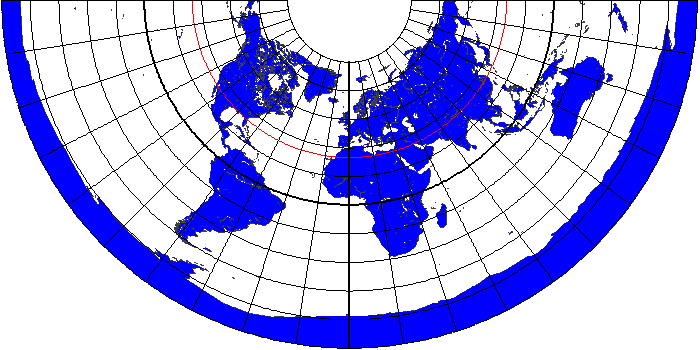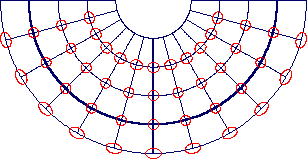×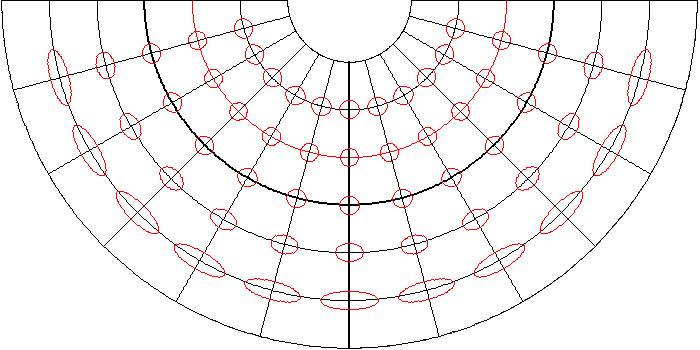×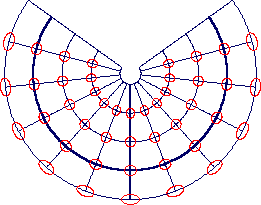×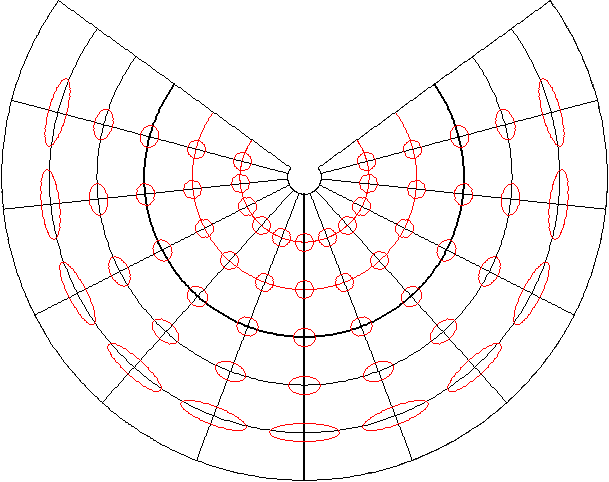Conformal conic projections (Tissot's Indicatrices with 30° graticule)×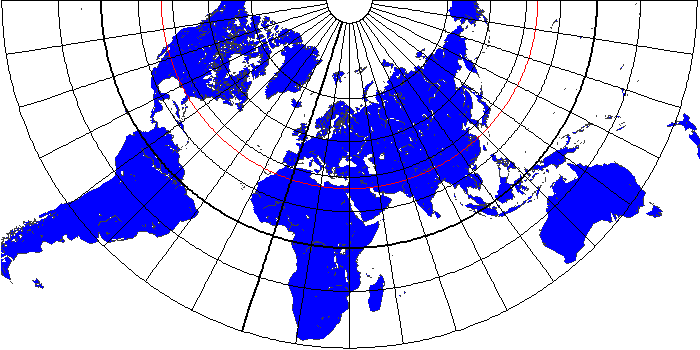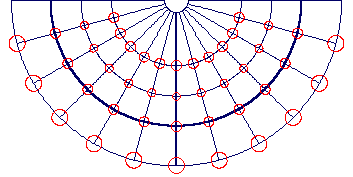×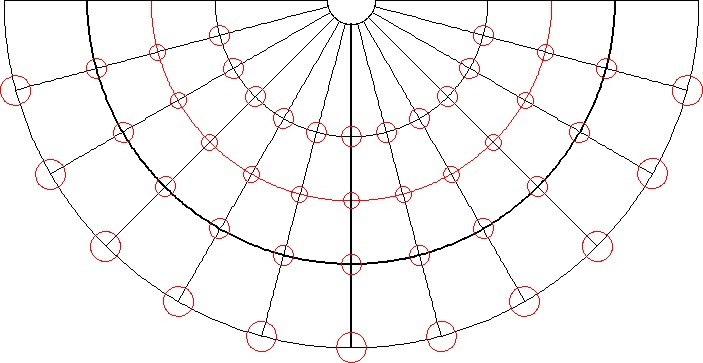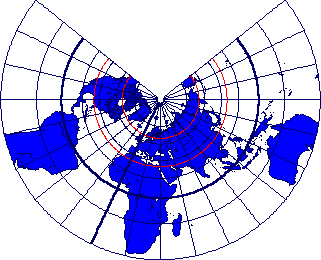×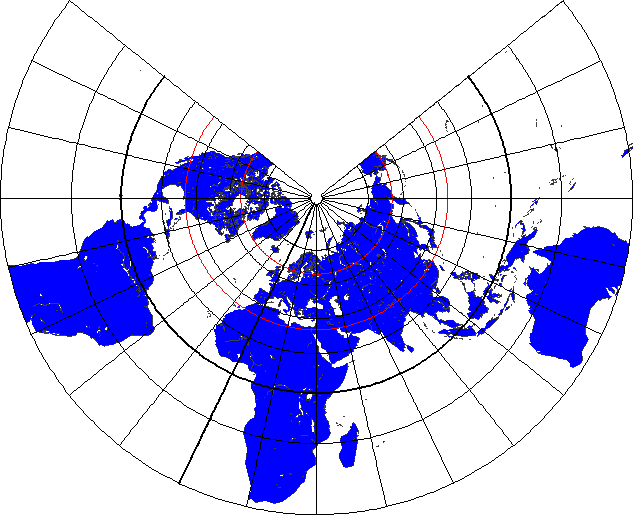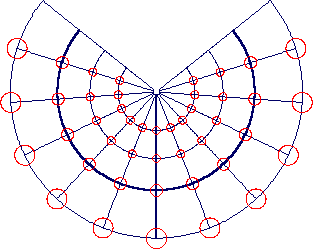×Lambert's equal-area conic projection (Tissot's Indicatrices with 30° graticule)×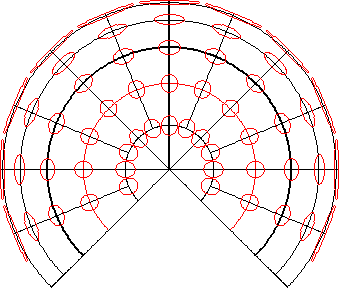×Azimuthal Projections

Equal-area azimuthal projections (Tissot's Indicatrices with 30° graticule)××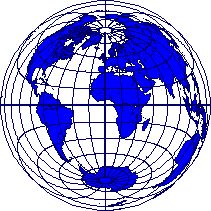×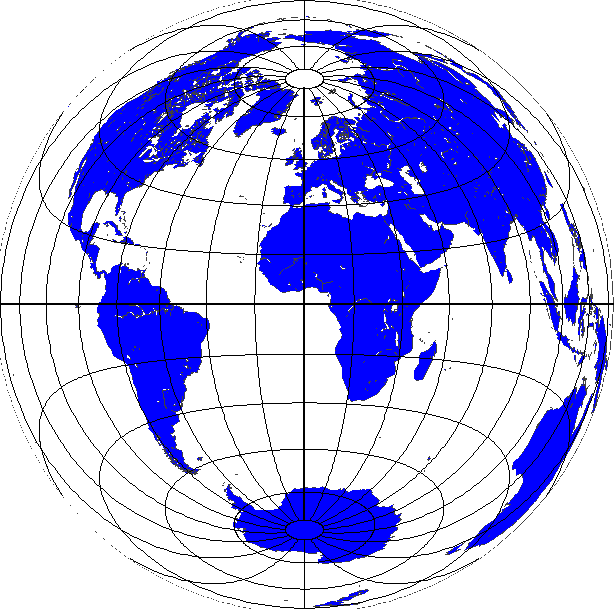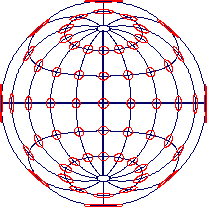×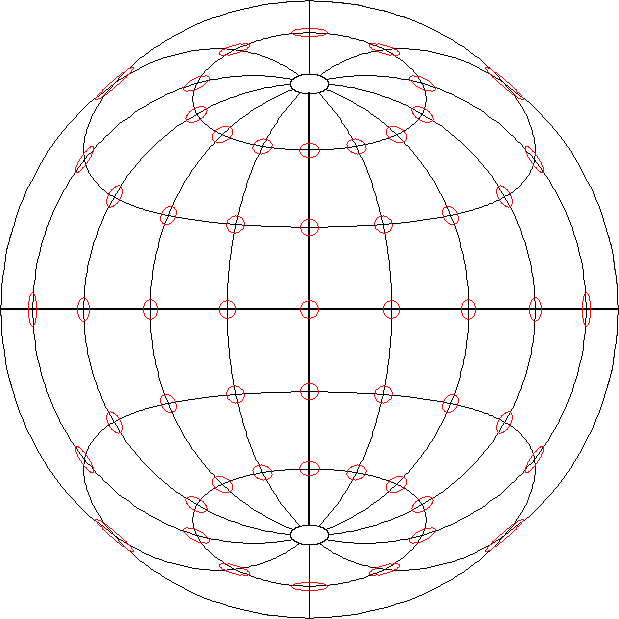Equidistant azimuthal projections (Tissot's Indicatrices with 30° graticule)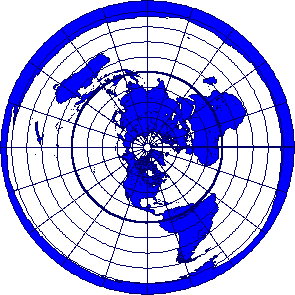×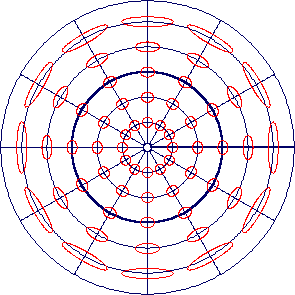×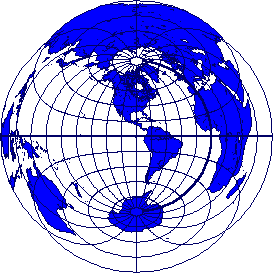×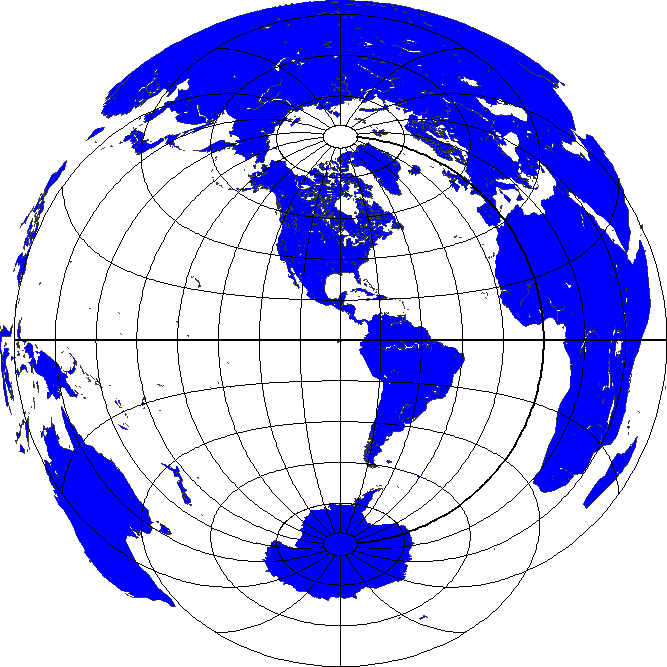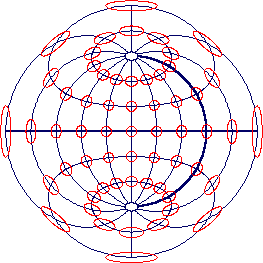×Conformal azimuthal projections (Tissot's Indicatrices with 30° graticule)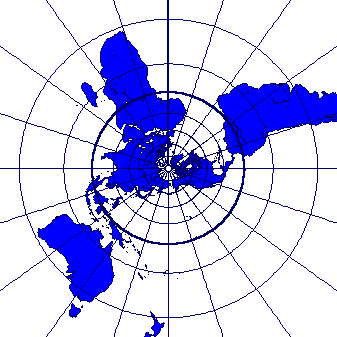××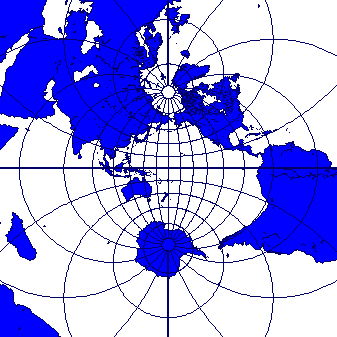×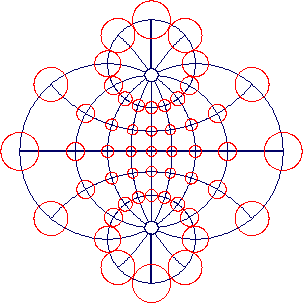×Orthographic projections (Tissot's Indicatrices with 30° graticule)×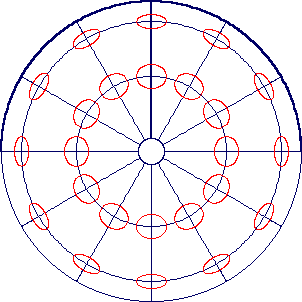×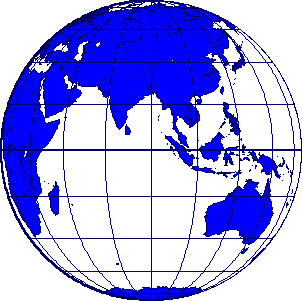×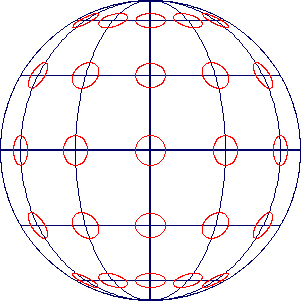×Gnomonic projections (Tissot's Indicatrices with 30° graticule)×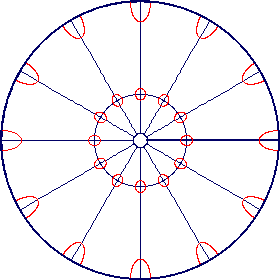×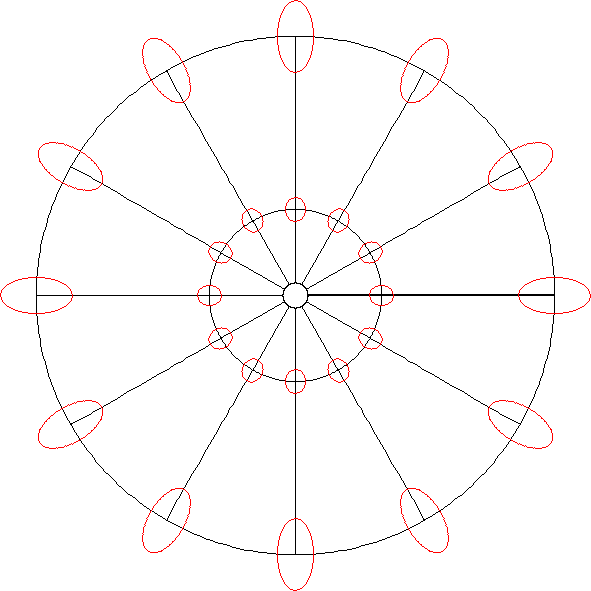××Cylindrical Projections

Equal-area cylindrical projections (Tissot's Indicatrices with 30° graticule)×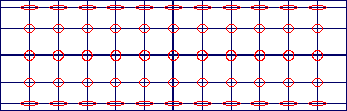×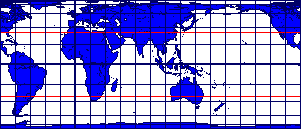×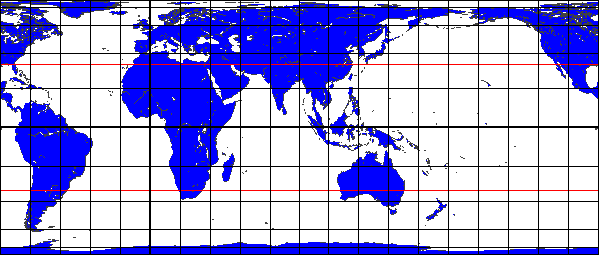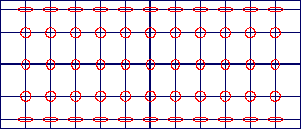×Equidistant cylindrical projections (Tissot's Indicatrices with 30° graticule)×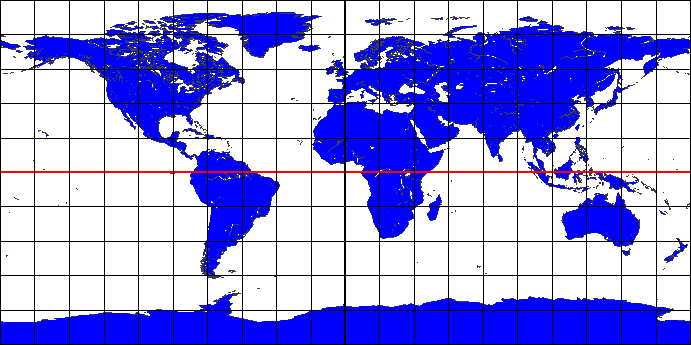×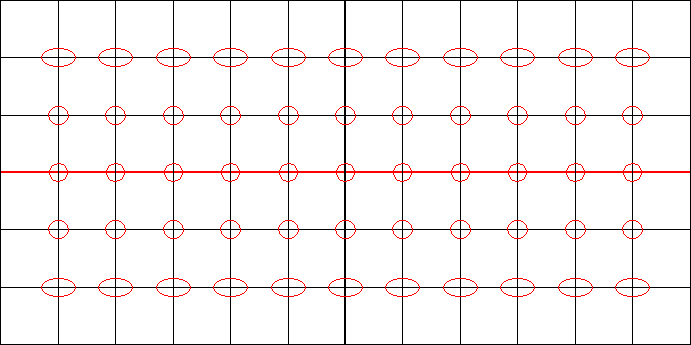×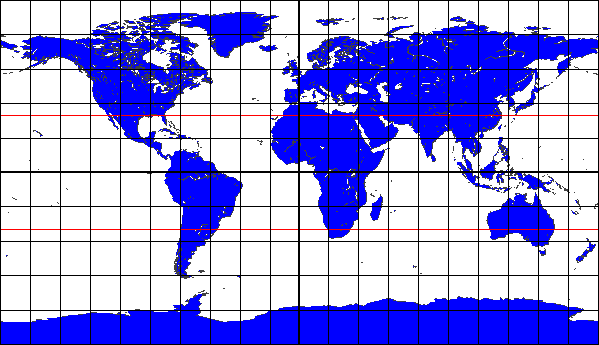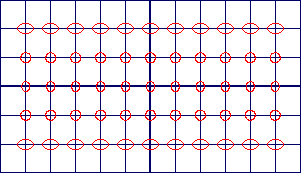×Conformal cylindrical projections (Tissot's Indicatrices with 30° graticule)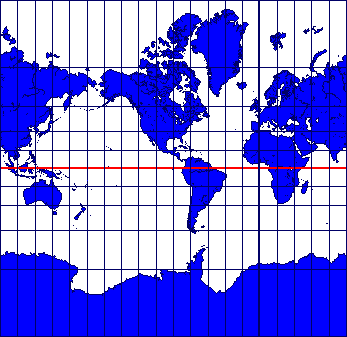××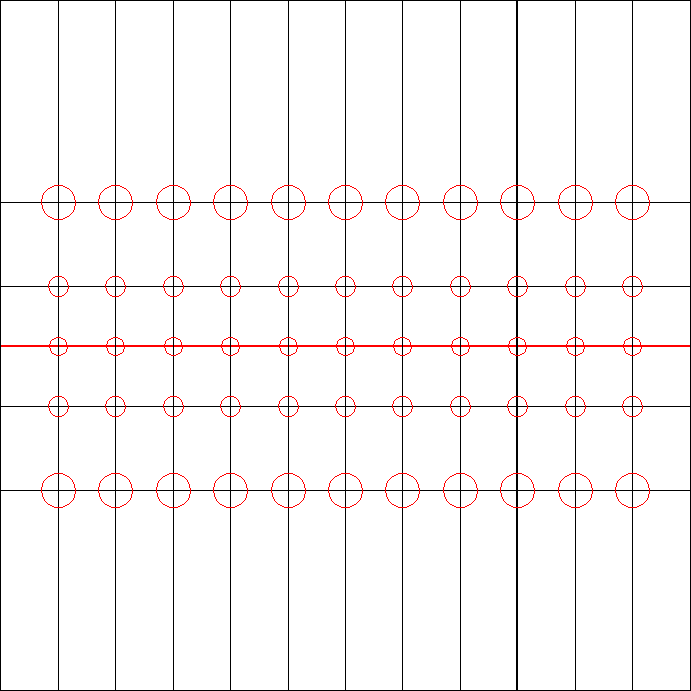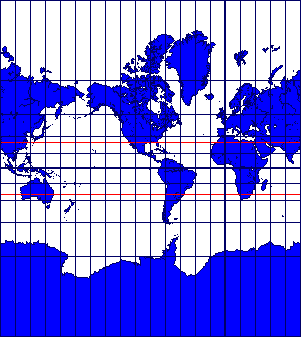×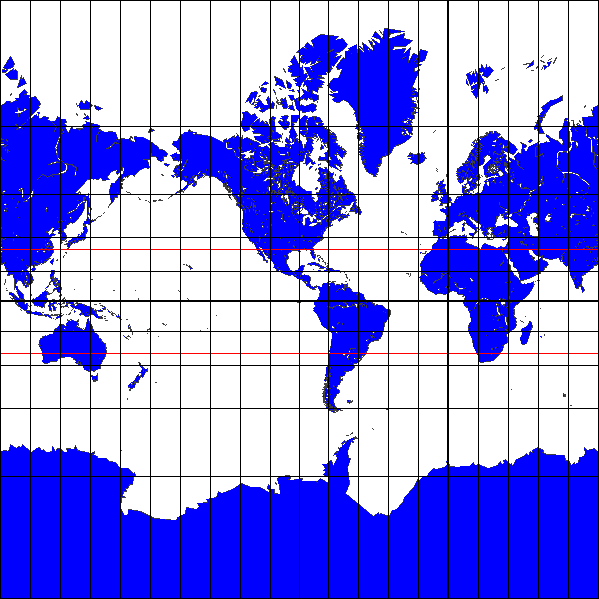×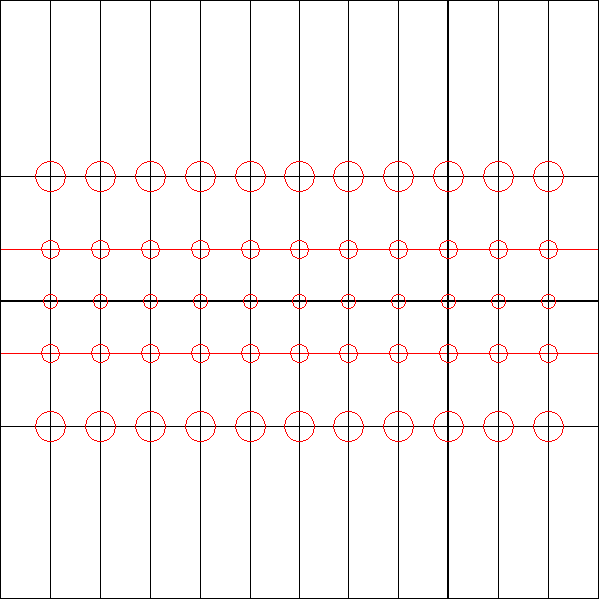Central perspective cylindrical projection (Tissot's Indicatrices with 30° graticule)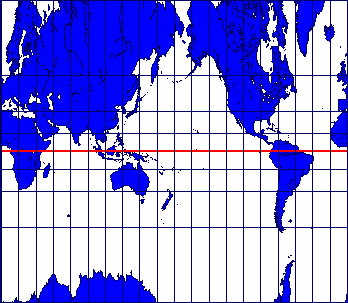×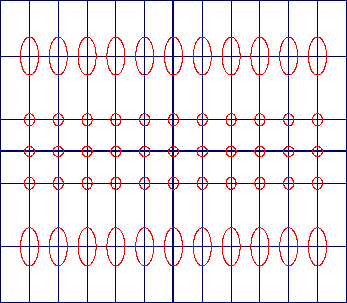×